# Buckets on a pulley - Dynamics Problem

• Shreya
In summary, the conversation discusses the calculation of initial velocity and momentum conservation in a system consisting of two buckets attached to a string and a putty. There is confusion about the given answer and the correct calculation of pre-impact velocity, as well as the consideration of the total mass of the system. Ultimately, the correct calculation is determined to be V=10/7 m/s, considering the signs of the momentum.

#### Shreya

Homework Statement
Two buckets of mass 6 kg are each attached to one end of a long inexten-
sible string passing over a fixed pulley. If a 2 kg mass of putty is dropped from a
height of 5 m into one bucket, calculate (i) the initial velocity of the system,
(ii) the acceleration of the system, (iii) the loss of energy of the 2 kg mass due to
the impact.
Relevant Equations
Newton's Laws
A) I am not sure if initial velocity means the initial velocity of the putty. If then, it should be 10m/s. But the answer given is 10/7 m/s
B) i have solved this part correctly
C) the answer should be 86J

Please be kind to help me

#### Attachments

•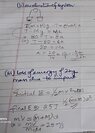20220416_095301.jpg
39.1 KB · Views: 100
Last edited:
Shreya said:
if initial velocity means iteh initial velocity of the putty
No it means the speeds of the three items (not velocities since the buckets move in opposite directions) immediately after the impact.

•MatinSAR and Shreya
The initial speeds (just before the collision) of both the buckets must be zero since they are of equal mass and of the putty would be 10m/s .The textbook gives the answer as 10/3 m/s. How do I get that?
haruspex said:
No it means the speeds of the three items (not velocities since the buckets move in opposite directions) immediately after the impact.

Shreya said:
The initial speeds (just before the collision) of both the buckets must be zero since they are of equal mass and of the putty would be 10m/s .The textbook gives the answer as 10/3 m/s. How do I get that?

•Shreya
Shreya said:
The initial speeds (just before the collision) of both the buckets must be zero since they are of equal mass and of the putty would be 10m/s .The textbook gives the answer as 10/3 m/s. How do I get that?
Post #1 shows that the answer given is 10/7 m/s.
Also, could you show how the pre-impact velocity of 10 m/s was calculated?

Lnewqban said:
Post #1 shows that the answer given is 10/7 m/s
I am sorry, 10/7 is the answer given by the book, not 10/3.
Lnewqban said:
show how the pre-impact velocity of 10 m/s was calculated?

I just used ##v=\sqrt{mgh}## to calculate the pre impact velocity of the putty

Last edited:
haruspex said:
after the collision,
Sorry, I missed that. By momentum conservation,
$$(M+m)V=mv$$
$$V= \frac{mv}{(M+m)}= 2.5 m/s$$

Shreya said:
Sorry, I missed that. By momentum conservation,
$$(M+m)V=mv$$
$$V= \frac{mv}{(M+m)}= 2.5 m/s$$
What does M represent?
Shouldn't it be the total mass of the system to which the 2 kg mass of putty became attached after the non-elastic collision?
Shouldn't that system be considered to be the two buckets of mass 6 kg each, attached to a common inextensible string?

Last edited:
Shreya said:
I am sorry, 10/7 is the answer given by the book, not 10/3.

I just used ##v=\sqrt{mgh}## to calculate the pre impact velocity of the putty
You should have used ##v=\sqrt{2gh}## for the pre impact speed.

•Shreya
Lnewqban said:
What does M represent?
Shouldn't it be the total mass of the system to which the 2 kg mass of putty became attached after the non-elastic collision?
Shouldn't that system be considered to be the two buckets of mass 6 kg each, attached to a common inextensible string?
Actually that is a mistake in the question. It's not whether it is inextensible, it’s whether it is inelastic.

•Shreya
haruspex said:
No it means the speeds of the three items (not velocities since the buckets move in opposite directions) immediately after the impact.
When calculating momentum just after collision, wouldn't the empty bucket have an upward momentum and the other bucket with putty a downward momentum? Therefore, the final momentum of pulley + putty system would be the difference of momentum of each bucket. I am considering the putty + both buckets as the system.
Maybe, I'm missing something here.

Last edited:
•Shreya
Sorry, I have been away for a few days.
I considered M as mass of one bucket, m as the mass of ball of putty, v as its velocity and V as the velocity of each bucket.
Lnewqban said:
Shouldn't it be the total mass of the system to which the 2 kg mass of putty became attached after the non-elastic collision

vcsharp2003 said:
Therefore, the final momentum of pulley + putty system would be the difference of momentum of each bucket. I am considering the putty + both buckets as the system

So would it be like this:
$$mv=(M+m)V + MV$$
$$V=\frac{mv}{2M+m}$$
$$V= \frac{10}{7}m/s$$

This has helped me solve part c also.
I also notice that I have not considered the signs in the above solution, yet got the correct answer. On applying the signs, I get ##v=V##. Could someone explain to me why this happens?

Shreya said:
. I also notice that I have not considered the signs in the above solution, yet got the correct answer.

The putty + bucket momentum is opposite in direction to momentum of the empty bucket, so shouldn't the RHS of your equation reflect this fact? Right now as per your equation, both the buckets are being treated as one object with mass ##2M## to which the putty sticks. Maybe because in connected system of masses we don't care about momentum directions of individual masses.

•Shreya
vcsharp2003 said:
The putty + bucket momentum is opposite in direction to momentum of the empty bucket, so shouldn't the RHS of your equation reflect this fact?
I agree. But on applying the signs, i get this:
##mv = (M+m)V - MV##
##v=V##, which doesn't seem right

Shreya said:
I agree. But on applying the signs, i get this:
##mv = (M+m)V - MV##
##v=V##, which doesn't seem right
Yes, but that still doesn't explain why the two buckets are treated as one mass for the purpose of getting total momentum?

•Shreya
vcsharp2003 said:
Yes, but that still doesn't explain why the two buckets are treated as one mass for the purpose of getting total momentum?
It’s just a useful ‘trick’. It works because the fixed pulley and inextensible string simply change the direction of the ‘connecting force’ between the buckets. In a suitably limited context, this means the two buckets (each mass M) can be treated as a single mass of 2M.

Note, during the collision (putty sticking to a bucket) the external supporting force (acting vertically upwards on the pulley) increases. A rigorous analysis (i.e. not using any ‘tricks’) would need to include the resulting external impulse, which sounds messy.

Edit - typo's corrected.

••Shreya, Lnewqban and vcsharp2003
Steve4Physics said:
It’s just a useful ‘trick’. It works because the fixed pulley and inextensible string simply change the direction of the ‘connecting force’ between the buckets. In a suitably limited context, this means the two buckets (each mass M) can be treated as a single mass of 2M.

Note, during the collision (putty sticking to a bucket) the external supporting force (acting vertically upwards on the pulley) increases. A rigorous analysis (i.e. not using any ‘tricks’) would need to include the resulting external impulse, which sounds messy.

Edit - typo's corrected.
It's still not clear, but I will read more and try to understand.

My reasoning is that any system can be broken down into parts and then the momentum calculated for each part. The system's momentum would then be the vector sum of momentum of individual parts.

•Shreya
Steve4Physics said:
It’s just a useful ‘trick’. It works because the fixed pulley and inextensible string simply change the direction of the ‘connecting force’ between the buckets. In a suitably limited context, this means the two buckets (each mass M) can be treated as a single mass of 2M.

Note, during the collision (putty sticking to a bucket) the external supporting force (acting vertically upwards on the pulley) increases. A rigorous analysis (i.e. not using any ‘tricks’) would need to include the resulting external impulse, which sounds messy.

Edit - typo's corrected.
I came up with some analysis that yields the conservation of momentum as is being applied in this scenario. Not sure if my assumption is correct related to the average force ##F_a## during the period when putty finally sticks to the bucket. I have assumed that this average force is going to be very large compared to ##mg## where ##m## is mass of putty. ##M## and ##V## represent the mass of each bucket and final speed of the system just after putty sticks to the bucket. ##v_i## is the velocity of putty just before the sticking process starts.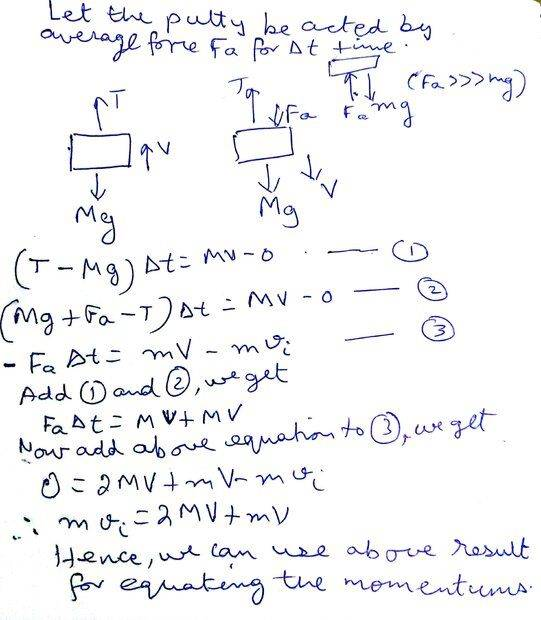•Steve4Physics and Shreya
vcsharp2003 said:
It's still not clear, but I will read more and try to understand.

My reasoning is that any system can be broken down into parts and then the momentum calculated for each part. The system's momentum would then be the vector sum of momentum of individual parts.
Also remember that a system's momentum can change if external force(s) act on it.

•vcsharp2003
vcsharp2003 said:
I came up with some analysis that yields the conservation of momentum as is being applied in this scenario. Not sure if my assumption is correct related to the average force ##F_a## during the period when putty finally sticks to the bucket. I have assumed that this average force is going to be very large compared to ##mg## where ##m## is mass of putty. ##M## and ##V## represent the mass of each bucket and final speed of the system just after putty sticks to the bucket. ##v_i## is the velocity of putty just before the sticking process starts.

View attachment 300345
Yes, that looks right.

•vcsharp2003
vcsharp2003 said:
I came up with some analysis that yields the conservation of momentum as is being applied in this scenario. Not sure if my assumption is correct related to the average force ##F_a## during the period when putty finally sticks to the bucket. I have assumed that this average force is going to be very large compared to ##mg## where ##m## is mass of putty. ##M## and ##V## represent the mass of each bucket and final speed of the system just after putty sticks to the bucket. ##v_i## is the velocity of putty just before the sticking process starts.

View attachment 300345
A few thoughts…

It is not clear if/where/how/why you have applied the assumption ##F_a>>mg##.

After the ‘collision’:
the momentum of the left bucket is ##MV## upwards (in vector notation ##MV \hat j##);
the momentum of the right bucket is ##MV## downwards (in vector notation ##-MV \hat j##).
Their vector sum is zero, not ‘2MV’.

However, your approach works because you have effectively used the ‘trick’ explained in Post #16! It's a work-around but not rigorous.

Also note, you have treated tension as if it is constant. But tension is different before and after the ‘collision’. I suppose you could say ##T## is the average tension during the collision.

•Shreya
Steve4Physics said:
Also note, you have treated tension as if it is constant. But tension is different before and after the ‘collision’. I suppose you could say ##T## is the average tension during the collision.
If you are going to skip the "trick" and go with the more rigorous treatment then the collision is an impulsive event and the average tension ##T## during the event is undefined. Instead, we would be interested in the integral of ##T## during the collision. That is, we would care about the impulse delivered by ##T##. Given the geometry of the ideal string and pulley, the delivered impulse would be identical on each bucket of course. (Equal, rather than equal and opposite).

That impulse would be whatever is required to maintain the constraint that the two buckets end up moving at equal and opposite velocities.

I do not recommend such an approach. It is sure to yield the same answer as the trick. The added complexity it not worth the effort. In my opinion anyway. Others are free to disagree.

••vcsharp2003, Steve4Physics and Shreya
Steve4Physics said:
It is not clear if/where/how/why you have applied the assumption
#18 has considered only the ##F_a## and not ##mg## when calculating the impulse for the putty. I don't understand why it is done so.

Shreya said:
#18 has considered only the ##F_a## and not ##mg## when calculating the impulse for the putty. I don't understand why it is done so.
The impulse delivered by force ##mg## over a time interval ##\Delta t## is ##mg \Delta t##. The shorter the time interval, the lower the delivered impulse. If the collision is very quick, the impulse from gravity during the collision becomes negligible.

••Steve4Physics, Shreya and vcsharp2003
•Lnewqban, Steve4Physics and vcsharp2003
Steve4Physics said:
It is not clear if/where/how/why you have applied the assumption Fa>>mg.
All I was trying to do was understand how the "trick" came to be valid in this scenario. Actually, I derived the so called "trick" using impulse equations. The "trick" is not truly reflecting the law of conservation of momentum as we are taught to apply it, so I drew inspiration from your post#16 which mentions using impulse equation as an alternate way of approaching this problem. After reading your post, I simply drew free body diagrams of each bucket and the piece of putty. I then applied the impulse equation to each free body diagram i.e. ## \vec F \Delta {t} = \Delta {\vec p}## and ended up with the equations numbered 1,2 and 3. In equation (3), I made an assumption that ##F_a >>> mg##, since in collisions this is a reasonable assumption as explained in @jbriggs444 post#24. Even if I don't assume this, we'll end up with an extra term ##mg\Delta{t}##, which we can ignore since ##\Delta {t}## is very, very small in a collision.

Steve4Physics said:
However, your approach works because you have effectively used the ‘trick’ explained in Post #16! It's a work-around but not rigorous.
I have not used the trick in post# 16, but actually derived the trick mentioned in that post. Look at the handwritten analysis I carried out in post#18 and look at the last equation that's derived using the three impulse equations for each object in this case. Each impulse equation boils down to an algebraic equation and then I simply add these algebraic equations to arrive at the final equation being used by the suggested trick. The last equation mentioned in my hand written analysis is the trick that was suggested for this scenario. But now I feel convinced why we can use the trick you suggested even though it appears counter-intuitive to the way law of conservation of momentum is applied using vector directions of each momentum quantity.

Steve4Physics said:
Also note, you have treated tension as if it is constant. But tension is different before and after the ‘collision’. I suppose you could say T is the average tension during the collision.
I could have taken definite integrals to reflect the varying nature of force of collision between putty/bucket and the tension, but it's easier to just use average force for each of these varying forces to make the 3 equations simpler looking; if we used the definite integrals, then the integrals would still cancel out during addition of impulse equations.

Steve4Physics said:
After the ‘collision’:
the momentum of the left bucket is MV upwards (in vector notation MVj^);
the momentum of the right bucket is MV downwards (in vector notation −MVj^).
Their vector sum is zero, not ‘2MV’.
I'm not adding momentums in my analysis but simply writing impulse equation for each object in the picture, so it doesn't matter what directions the momentums of the two buckets are in as long as I write the correct impulse equation for each bucket. In this situation, the empty bucket is assumed to move up and putty bucket is assumed to move down, and equations 1 and 2 in my analysis are consistent with these directions.

Actually, if I don't assume that ##F_a >>> mg##, I would end up with the equation below, which clearly means that the "trick" is an approximation where we ignored the second term on LHS.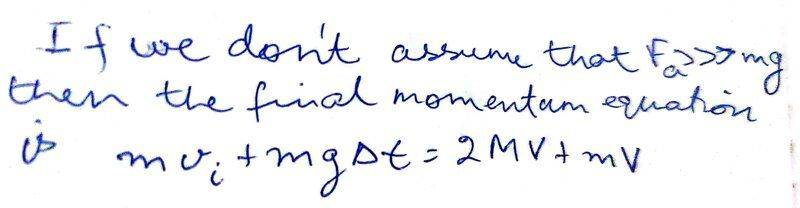Last edited:
•Shreya
vcsharp2003 said:
I'm not adding momentums in my analysis but simply writing impulse equation for each object in the picture, so it doesn't matter what directions the momentums of the two buckets are in as long as I write the correct impulse equation for each bucket.
I will add this if I may.

In your Post #18 attachment you have, for the two buckets:
##(T – Mg)\Delta t = MV – 0## (equation 1)
##(Mg + F_a – T)\Delta t = MV – 0## (equation 2)

Equation 1 uses upwards as positive and equation 2 uses downwards as positive.

In general, you can't combine vector-equations where different sign-conventions have been used in the different equations.

However (because of the pulley and the inextensible string) it turns out that, in this particular situation, the directions don’t matter. It is OK, for example, to add your two equations giving. ##F_a \Delta t = 2MV## (remembering ##V## is speed, not velocity).

Note that ##2MV## is not the total momentum of the buckets. The total momentum of the buckets has magnitude ##MV+M(-V) = 0##. (In fact the total momentum of the buckets is always zero.)

This then leads to an interesting question:

If an impulse of ##F_a \Delta t## has been applied, how come the total momentum of the buckets is zero?!

•Shreya
Steve4Physics said:
In general, you can't combine vector-equations where different sign-conventions have been used in the different equations.
In my view, we are just equating the magnitudes in each impulse equation to get a simple algebraic equation, and therefore, it shouldn't matter what +ve direction we take for each bucket.

On the other hand, if one wants to stick to the same +ve direction for all masses, then also we get equations that when subtracted from each other give the same result. I have verified this, but I believe we can take a different +ve direction for each since we are talking about relationship between magnitudes in each impulse equation.
Steve4Physics said:
If an impulse of FaΔt has been applied, how come the total momentum of the buckets is zero?!
The total impulse on each bucket is not just due to ##F_a## but tension and weight need to have their impulses also included when looking at each mass. The impulse equation for each mass clearly tells us the momentum change with the constraint that speed of the two buckets is same just after collision.

•Steve4Physics
vcsharp2003 said:
In my view, we are just equating the magnitudes in each impulse equation to get a simple algebraic equation, and therefore, it shouldn't matter what +ve direction we take for each bucket.
Agreed. That's a good way to look at it.

vcsharp2003 said:
On the other hand, if one wants to stick to the same +ve direction for all masses, then also we get equations that when subtracted from each other give the same result. I have verified this, but I believe we can take a different +ve direction for each since we are talking about relationship between magnitudes in each impulse equation.
Yes. If using magnitudes and the correct relative directions (the '+'s and '-'s) it shouldn't matter which direction you take as +ve.

vcsharp2003 said:
The total impulse on each bucket is not just due to ##F_a## but tension and weight need to have their impulses also included when looking at each mass. The impulse equation for each mass clearly tells us the momentum change with the constraint that speed of the two buckets is same just after collision.

Just before impact, the system (buckets + putty) has total momentum ##mv##.
Just after impact, the system has total momentum ##mV## (because the buckets have zero total momentum due to their opposite velocities).

I wanted to pose the question (mainly for the OP's benefit): ‘How was the missing momentum (##mv-mV##) lost?’.

•Shreya
After the putty is stuck to the bucket, this becomes an Atwood machine situation. The so called trick used here is essentially the same as the one used in writing down the equation of motion for the Atwood machine. The argument is that since the tension is internal and the massless pulley just changes the direction of the tension but not its magnitude, one can "straighten out" the string and consider the dynamically equivalent situation in which two masses are connected with a massless rigid rod (replacing the string to avoid questions of compression) on a frictionless horizontal table with force ##F_1=m_1g## pulling to the left on ##m_1## and ##F_2=m_2g## pulling to the right on ##m_2##. Then $$m_2g-m_1g=(m_1+m_2)a\implies a=\frac{m_2-m_1}{m_2+m_1}g.$$Here, we have a similar picture. Consider first two masses ##m_1 =m_2=M## connected with a light rod on a horizontal frictionless plane with equal forces ##F_1=F_2=Mg## pulling to the left and to the right respectively. A third mass ##m_3=m## moving to the right is pulled with force ##F_3=mg## to the right. When its velocity is ##v## parallel to the rod, it collides and sticks to ##m_2##. Assuming that the collision is instantaneous, the final velocity of the composite system is obtained from momentum conservation, $$mv=(m+M+M)V\implies V=\frac{mv}{m+2M}.$$Now if we left the system alone on the table, it will keep on sliding forever with the masses having common acceleration $$a=\frac{F_2+F_3-F_1}{m+2M}=\frac{(M+m)g-Mg}{m+2M}=\frac{mg}{m+2M}.$$ If, however, we unstraighten the rod and change it to two vertical strings, we will have an Atwood machine with masses ##m_1=M## and ##m_2=m+M##. Using the Atwood machine formula, we get the magnitude of the acceleration of the masses, $$a=\frac{m_2-m_1}{m_2+m_1}g=\frac{m+M-M}{m+M+M}g=\frac{mg}{m+2M}.$$We have consistency. The "straightening trick" helps sort out the instantaneous momentum conservation from the dynamics of this situation and works both ways, from Atwood machine to linear system and back.

Last edited:
•Steve4Physics, vcsharp2003, Shreya and 1 other person
Steve4Physics said:
I wanted to pose the question (mainly for the OP's benefit): ‘How was the missing momentum (mv−mV) lost?’
My guess is that due to collision of the putty of mass ##m## with bucket, the velocity of the putty decreases as a very large force ##F_a## acts upward on the putty for a very short duration which causes deceleration of the putty. Therefore, ##V < v## and so ##mV< mv## i.e final momentum is less than initial momentum for the mass ##m##.

Also, we could say that looking at the putty ##m## during its impact with the bucket, the putty probably gets deformed which means a part of putty's original KE gets partly converted to PE of deformed putty. Also, some of it's original KE is converted to sound and heat energy on impact. This means that kinetic energy of putty just after impact is less than its kinetic energy just before impact and therefore, its final velocity is less than its initial velocity making the putty lose some of it's momentum.

Last edited:
kuruman said:
We have consistency. The "straightening trick" helps sort out the instantaneous momentum conservation from the dynamics of this situation and works both ways, from Atwood machine to linear system and back.
That's a nice trick that helps to solve problems on Atwood machine. But I was wondering if it has a wider applicability when considering masses connected via pulleys like on an incline or more complex cases of Atwood machine as shown in images below. If it could, then this would be an awesome trick in solving many difficult problems.

I tried to apply your straightening trick to first pulley masses system and came up with following straightened string.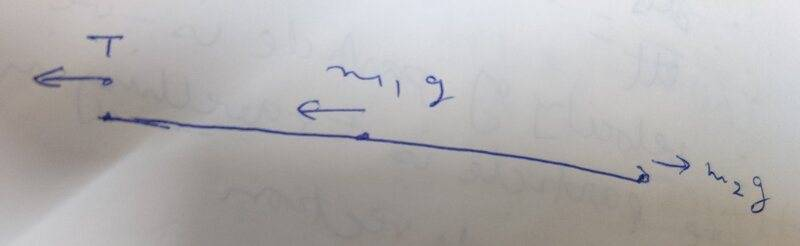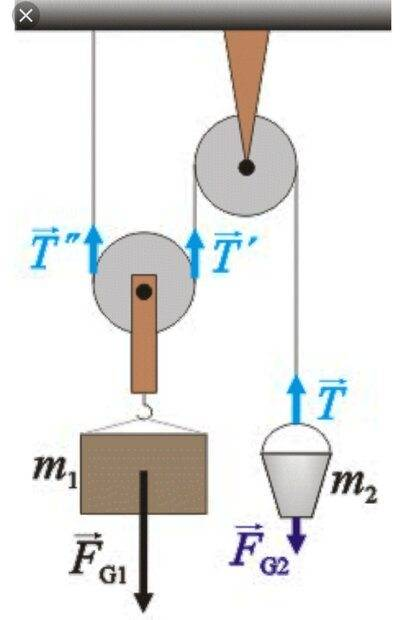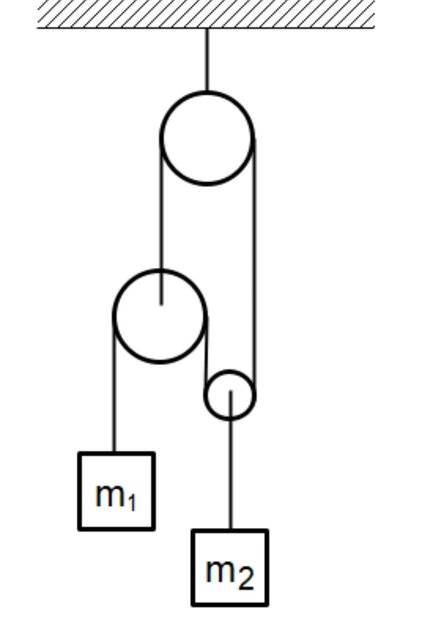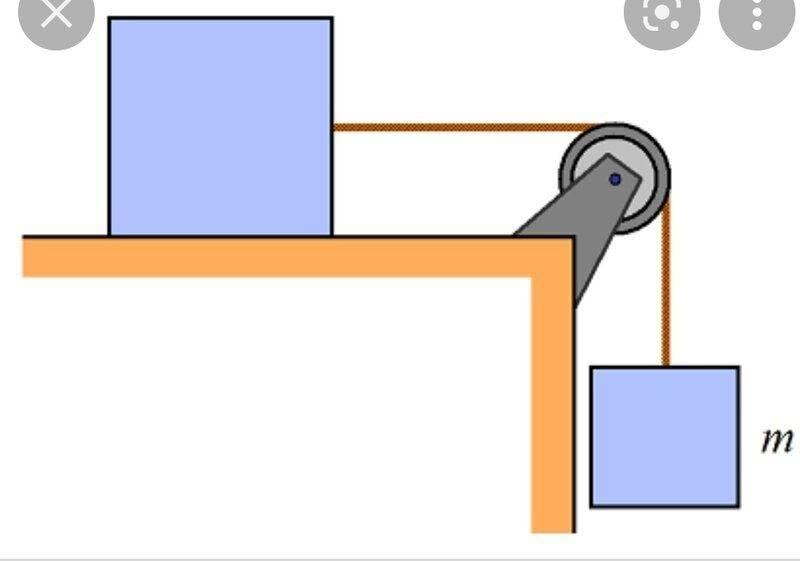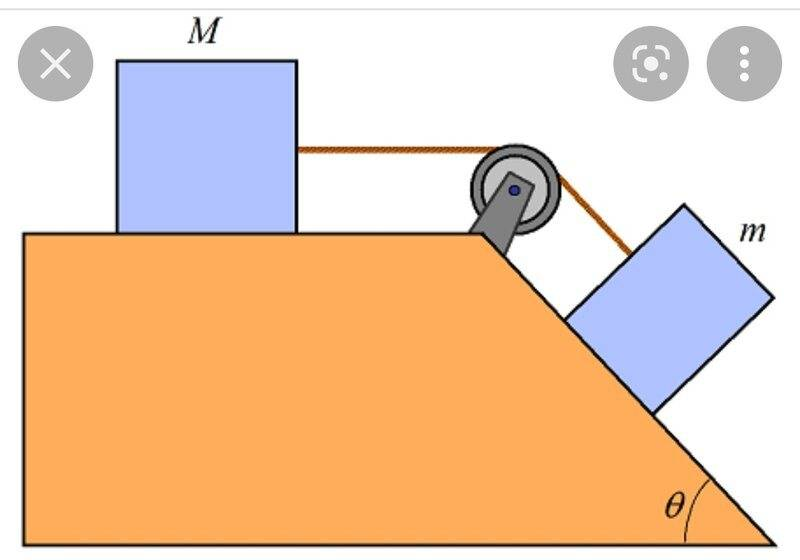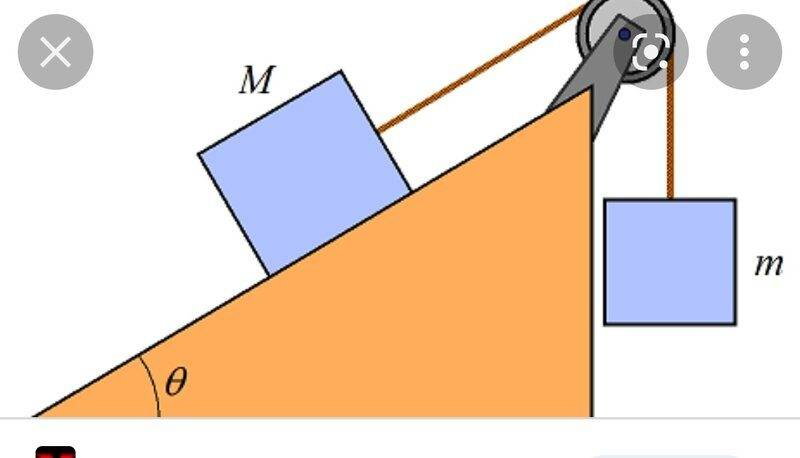Last edited:
vcsharp2003 said:
My guess is that due to collision of the putty of mass ##m## with bucket, the velocity of the putty decreases as a very large force ##F_a## acts upward on the putty for a very short duration which causes deceleration of the putty.
From Newton's 3rd law, another very large force ##F_a'## acts downwards on the bucket. ##F_a = -F_a'##. ##F_a## and ##F_a'## are internal forces in the system with a zero sum, so can't change the system's momentum.

vcsharp2003 said:
This means that kinetic energy of putty just after impact is less than its kinetic energy just before impact and therefore, its final velocity is less than its initial velocity making the putty lose some of it's momentum.
The interesting question is: where has the putty's lost momentum gone?

(Hint: see end of Post #16.)

Steve4Physics said:
so can't change the system's momentum.
But I was looking only at the mass of putty since you metioned ##mV - mv## as change in momentum and surely the impact force on this mass will decrease its speed, which will decrease it's momentum. The change in momentum equation is only depending on initial and final momentum of putty, so I focused only on the mass of putty.

Steve4Physics said:
Hint: see end of Post #16
All I see at end of post is that the supporting force of pulley increases during impact, but still I can't figure out how it relates to lost momentum. Maybe, if pulley + string + buckets + putty is considered as one system then the upward support force on pulley will exert an upward impulse versus downward impluse of weight of buckets and putty; the net impulse on this system would be upward which will mean the initial downward momentum of system will decrease.

•Steve4Physics